# Texas Go Math Grade 3 Lesson 15.4 Answer Key Identify Congruent Figures

Refer to our Texas Go Math Grade 3 Answer Key Pdf to score good marks in the exams. Test yourself by practicing the problems from Texas Go Math Grade 3 Lesson 15.4 Answer Key Identify Congruent Figures.

## Texas Go Math Grade 3 Lesson 15.4 Answer Key Identify Congruent Figures

Unlock the Problem

Figures that have the same size and the same shape are congruent.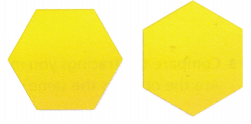Look at the pattern blocks at the right. Do the shapes appear to be congruent?

Compare size and shape.

These pairs of shapes appear to be congruent.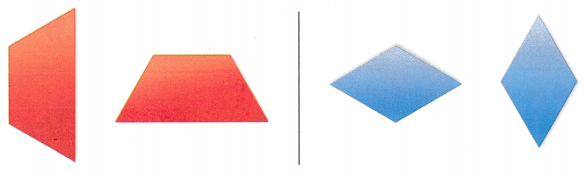Same size, same shape

These pairs of shapes are not congruent.The yellow pattern blocks appear to be __________size and _________ shape. So, they are _________.
The yellow pattern blocks appear to be same size and same shape. So, they are Congruent

Activity

Materials; pattern blocks, paper

Trace pattern blocks to find congruent figures.
STEP 1:
Trace a blue rhombus pattern block in the space below.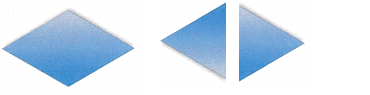STEP 2:
Trace two pattern blocks to make a figure that is congruent to the rhombus.STEP 3:
Are the outlines the same size and shape? ___________
Yes, The outlines are same size and shape

Are the figures you traced congruent? Explain.
Yes they are congruent.

Share and Show

Question 1.
Which pattern block appears to be congruent to Shape A?Think: Which pattern block is the same size and shape?
Explanation:
They both are triangles with equal sides

Circle the 2 pattern blocks you can combine to make a figure congruent to the hexagon.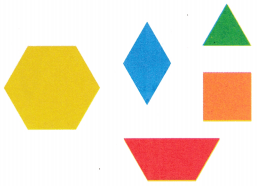2 rhombuses
2 squares
2 trapezoids
2 triangles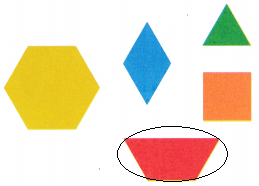Explanation:
2 trapezoids combine to make a figure congruent to the hexagon.

Math Talk
Mathematical Processes

For Exercise 1, a student said neither shape B or C is congruent to shape A. Explain why the student might think this.
Answer: C is in different direction.
Explanation:
a student said neither shape B or C is congruent to shape A.
The student might think that the B and C are not congruent because C in different direction.

Look at the first figure. Tell if it appears to be congruent to the second figure. Write yes or no.

Question 3.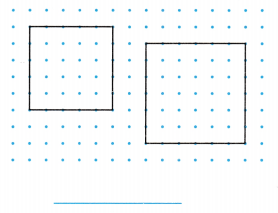Explanation:
Shapes are same but sizes are different

Question 4.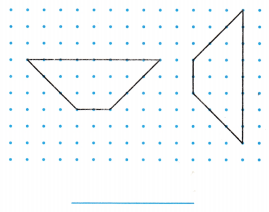Explanation:
The both figures have same size and shape. so, they are congruent.

Problem Solving

Question 5.
H.O.T. Multi-Step List all the ways you can use congruent pattern blocks to make the hexagon shown.Explanation:
The above picture shows the ways we can use congruent pattern blocks to make the hexagon

Write Math What’s the Error? Isabel says that all squares are congruent. Use Math Language to explain her error.
Answer: No, there must be size differ
Explanation:
Shape the square is common but, the size varies.

Question 7.
Use Diagrams List all the pairs of figures that appear to be congruent.W and Z are congruent
V and x are congruent
Explanation:
They have size and shape are equal.

Fill in the bubble for the correct answer choice.

Question 8.
Look at the kaleidoscope image and find the figure outlined in red. Which figure below appears to be congruent to it?(A) K
(B) L
(C) J
(D) M
Explanation:
The image L matches the shape
both are rhombus

Question 9.
Multi-Step Israel is making a pattern with quadrilaterals for a wallpaper border. Which figures appear to be congruent?(A) the two rectangles
(B) the trapezoid and one rectangle
(C) the square and one rectangle
(D) the rhombus and the square
Explanation:
Green and purple rectangles are congruent.

Texas Test Prep

Question 10.
Which pattern blocks can you combine to make a shape that is congruent to the shape below?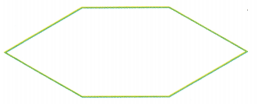(A) trapezoid and 2 triangles
(B) trapezoid and rhombus
(C) square and 2 triangles
(D) 2 rhombuses
Explanation:
square and 2 triangles combine to make a shape that is congruent to the  given shape

### Texas Go Math Grade 3 Lesson 15.4 Homework and Practice Answer Key

Look at the first figure. Tell if it appears to be congruent to the second figure. Write yes or no.

Question 1.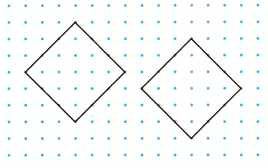Explanation:
The above figures are congruent
they have size and shapes are same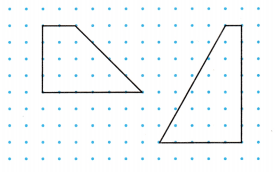Explanation:
Both the trapezoids are not same

Problem Solving

Question 3.
List all the ways you can use congruent pattern blocks to make the figure shown.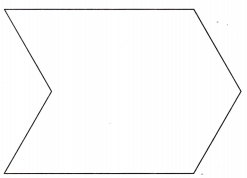Explanation:
The ways to make a congruent figure are done

Question 4.
List the pairs of figures that appear to be congruent.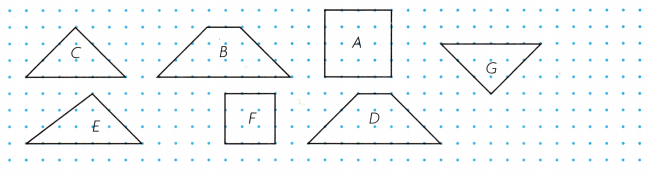C and G are congruent
B and D are Congruent
Explanation:
C and G , B and D have same sizes and same shape

Question 5.
Draw a congruent figure on the dot paper for each figure you did not list in exercise 4.Explanation:
Drawn a congruent figures for the Exercise4 which are not done.

Lesson Check

Question 6.
Ursula drew the congruent figures below. Which 2 pattern blocks can she combine to make a rhombus?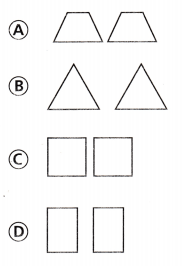Explanation:
The two triangles make a rhombus

Go Math Grade 3 Pdf Congruent Figures Question 7.
Albert wants to make a parallelogram. Which figures can he add to the ends of a square to make the shape?Explanation:
The shapes in B are added to a square to make a parallelogram

Question 8.
Lionel made some cutout shapes. Which figures do NOT appear to be congruent?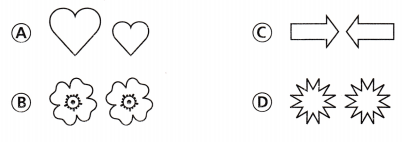Explanation:
Option A have same shape but different size

Texas Go Math Grade 3 Answer Key Pdf Identify Congruent Figures Question 9.
Multi-Step Manny drew the figure shown. What is the least number of pattern blocks he can use to make a congruent figure?(A) 2
(B) 5
(C) 5
(D) 3